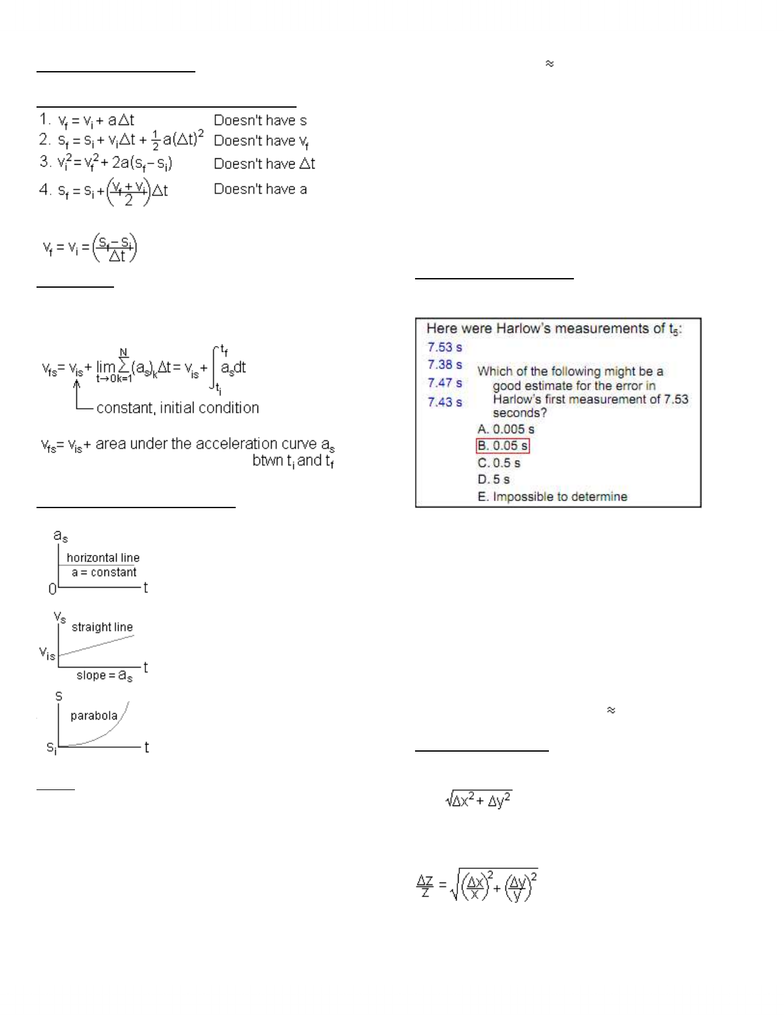Class Notes (1,100,000)
CA (650,000)
UTSG (50,000)
Physics (400)
Lecture 2

# Lecture 2 - Motion + Error Analysis, in 2 columns for easy printing+reading, includes diagrams I drew on the computer

Department
Physics
Course Code
PHY131H1
Professor
Jason Harlow
Lecture
2

This preview shows half of the first page. to view the full 3 pages of the document.PHY131H1 Summer Lecture #2
Error Analysis + Motion
The 4 Equations of Constant Acceleration
For zero acceleration:
Acceleration
-Can be negative, zero, or positive if the object is
stopped (even momentarily)
-SI unit = m/s per second = m/s2
Graphically:
Motion at Constant Acceleration
Ex. Free-fall, projectiles (ignore air resistance)
Errors
Rules: 1) Quote errors to 1 or 2 sigfigs
2) Make sure both number and error have the
same # of digits after the decimal place
(Basically, make sure they both end in the
same column, ex. Hundredths)
3) For other numbers, assume the error is
half the value of the last column
(ex. Error = 0.005 if the last column of the
number is the hundredths)
-Eliminate need to report measurements w/
approximately /
-Give quantitative way or stating your confidence
level in the measurement
o = error
o68% sure the actual # is btwn and + 
o95% sure the actual # is btwn-2 and +2 
Ex. Answer = 10 ± 2
o68% sure the actual # is btwn 8 and 12
o95% sure the actual # is btwn 6 and 14
Ex. Period of a Pendulum
-t5 = time for 5 oscillations
-Period = t5÷5
-A. Would be error if:
oSmaller scatter
oOnly 1 measurement taken
oOr if each measurement was 7.53_s, but
the stopwatch only shows up to the
hundredth place
-B. Is the best estimate for the error, since:
oIs almost half the range of the
measurements,
oAnd is for repeated measurements
-t5, avg = 7.45250s
-est = 0.0634429s = 0.06s A ÷ 83
Propagation of Errors
Sum/Difference Rules:
If z = x + y or z = x y
z =
Product/Division Rules:
If z = x*y or z = x / y
If z = A*x
z = Ax
Exponent Rule:
www.notesolution.com